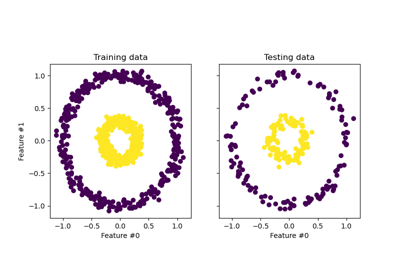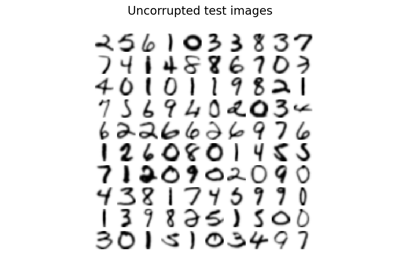# sklearn.decomposition.KernelPCA¶

class sklearn.decomposition.KernelPCA(n_components=None, *, kernel='linear', gamma=None, degree=3, coef0=1, kernel_params=None, alpha=1.0, fit_inverse_transform=False, eigen_solver='auto', tol=0, max_iter=None, iterated_power='auto', remove_zero_eig=False, random_state=None, copy_X=True, n_jobs=None)[source]

Kernel Principal component analysis (KPCA) .

Non-linear dimensionality reduction through the use of kernels (see Pairwise metrics, Affinities and Kernels).

It uses the scipy.linalg.eigh LAPACK implementation of the full SVD or the scipy.sparse.linalg.eigsh ARPACK implementation of the truncated SVD, depending on the shape of the input data and the number of components to extract. It can also use a randomized truncated SVD by the method proposed in , see eigen_solver.

Read more in the User Guide.

Parameters:
n_componentsint, default=None

Number of components. If None, all non-zero components are kept.

kernel{‘linear’, ‘poly’, ‘rbf’, ‘sigmoid’, ‘cosine’, ‘precomputed’} or callable, default=’linear’

Kernel used for PCA.

gammafloat, default=None

Kernel coefficient for rbf, poly and sigmoid kernels. Ignored by other kernels. If gamma is None, then it is set to 1/n_features.

degreeint, default=3

Degree for poly kernels. Ignored by other kernels.

coef0float, default=1

Independent term in poly and sigmoid kernels. Ignored by other kernels.

kernel_paramsdict, default=None

Parameters (keyword arguments) and values for kernel passed as callable object. Ignored by other kernels.

alphafloat, default=1.0

Hyperparameter of the ridge regression that learns the inverse transform (when fit_inverse_transform=True).

fit_inverse_transformbool, default=False

Learn the inverse transform for non-precomputed kernels (i.e. learn to find the pre-image of a point). This method is based on .

eigen_solver{‘auto’, ‘dense’, ‘arpack’, ‘randomized’}, default=’auto’

Select eigensolver to use. If n_components is much less than the number of training samples, randomized (or arpack to a smaller extent) may be more efficient than the dense eigensolver. Randomized SVD is performed according to the method of Halko et al .

auto :

the solver is selected by a default policy based on n_samples (the number of training samples) and n_components: if the number of components to extract is less than 10 (strict) and the number of samples is more than 200 (strict), the ‘arpack’ method is enabled. Otherwise the exact full eigenvalue decomposition is computed and optionally truncated afterwards (‘dense’ method).

dense :

run exact full eigenvalue decomposition calling the standard LAPACK solver via scipy.linalg.eigh, and select the components by postprocessing

arpack :

run SVD truncated to n_components calling ARPACK solver using scipy.sparse.linalg.eigsh. It requires strictly 0 < n_components < n_samples

randomized :

run randomized SVD by the method of Halko et al. . The current implementation selects eigenvalues based on their module; therefore using this method can lead to unexpected results if the kernel is not positive semi-definite. See also .

Changed in version 1.0: 'randomized' was added.

tolfloat, default=0

Convergence tolerance for arpack. If 0, optimal value will be chosen by arpack.

max_iterint, default=None

Maximum number of iterations for arpack. If None, optimal value will be chosen by arpack.

iterated_powerint >= 0, or ‘auto’, default=’auto’

Number of iterations for the power method computed by svd_solver == ‘randomized’. When ‘auto’, it is set to 7 when n_components < 0.1 * min(X.shape), other it is set to 4.

New in version 1.0.

remove_zero_eigbool, default=False

If True, then all components with zero eigenvalues are removed, so that the number of components in the output may be < n_components (and sometimes even zero due to numerical instability). When n_components is None, this parameter is ignored and components with zero eigenvalues are removed regardless.

random_stateint, RandomState instance or None, default=None

Used when eigen_solver == ‘arpack’ or ‘randomized’. Pass an int for reproducible results across multiple function calls. See Glossary.

New in version 0.18.

copy_Xbool, default=True

If True, input X is copied and stored by the model in the X_fit_ attribute. If no further changes will be done to X, setting copy_X=False saves memory by storing a reference.

New in version 0.18.

n_jobsint, default=None

The number of parallel jobs to run. None means 1 unless in a joblib.parallel_backend context. -1 means using all processors. See Glossary for more details.

New in version 0.18.

Attributes:
eigenvalues_ndarray of shape (n_components,)

Eigenvalues of the centered kernel matrix in decreasing order. If n_components and remove_zero_eig are not set, then all values are stored.

eigenvectors_ndarray of shape (n_samples, n_components)

Eigenvectors of the centered kernel matrix. If n_components and remove_zero_eig are not set, then all components are stored.

dual_coef_ndarray of shape (n_samples, n_features)

Inverse transform matrix. Only available when fit_inverse_transform is True.

X_transformed_fit_ndarray of shape (n_samples, n_components)

Projection of the fitted data on the kernel principal components. Only available when fit_inverse_transform is True.

X_fit_ndarray of shape (n_samples, n_features)

The data used to fit the model. If copy_X=False, then X_fit_ is a reference. This attribute is used for the calls to transform.

n_features_in_int

Number of features seen during fit.

New in version 0.24.

feature_names_in_ndarray of shape (n_features_in_,)

Names of features seen during fit. Defined only when X has feature names that are all strings.

New in version 1.0.

FastICA

A fast algorithm for Independent Component Analysis.

IncrementalPCA

Incremental Principal Component Analysis.

NMF

Non-Negative Matrix Factorization.

PCA

Principal Component Analysis.

SparsePCA

Sparse Principal Component Analysis.

TruncatedSVD

Dimensionality reduction using truncated SVD.

References

Examples

>>> from sklearn.datasets import load_digits
>>> from sklearn.decomposition import KernelPCA
>>> transformer = KernelPCA(n_components=7, kernel='linear')
>>> X_transformed = transformer.fit_transform(X)
>>> X_transformed.shape
(1797, 7)


Methods

 fit(X[, y]) Fit the model from data in X. fit_transform(X[, y]) Fit the model from data in X and transform X. get_feature_names_out([input_features]) Get output feature names for transformation. get_params([deep]) Get parameters for this estimator. Transform X back to original space. set_output(*[, transform]) Set output container. set_params(**params) Set the parameters of this estimator. Transform X.
fit(X, y=None)[source]

Fit the model from data in X.

Parameters:
X{array-like, sparse matrix} of shape (n_samples, n_features)

Training vector, where n_samples is the number of samples and n_features is the number of features.

yIgnored

Not used, present for API consistency by convention.

Returns:
selfobject

Returns the instance itself.

fit_transform(X, y=None, **params)[source]

Fit the model from data in X and transform X.

Parameters:
X{array-like, sparse matrix} of shape (n_samples, n_features)

Training vector, where n_samples is the number of samples and n_features is the number of features.

yIgnored

Not used, present for API consistency by convention.

**paramskwargs

Parameters (keyword arguments) and values passed to the fit_transform instance.

Returns:
X_newndarray of shape (n_samples, n_components)

Returns the instance itself.

get_feature_names_out(input_features=None)[source]

Get output feature names for transformation.

The feature names out will prefixed by the lowercased class name. For example, if the transformer outputs 3 features, then the feature names out are: ["class_name0", "class_name1", "class_name2"].

Parameters:
input_featuresarray-like of str or None, default=None

Only used to validate feature names with the names seen in fit.

Returns:
feature_names_outndarray of str objects

Transformed feature names.

get_params(deep=True)[source]

Get parameters for this estimator.

Parameters:
deepbool, default=True

If True, will return the parameters for this estimator and contained subobjects that are estimators.

Returns:
paramsdict

Parameter names mapped to their values.

inverse_transform(X)[source]

Transform X back to original space.

inverse_transform approximates the inverse transformation using a learned pre-image. The pre-image is learned by kernel ridge regression of the original data on their low-dimensional representation vectors.

Note

When users want to compute inverse transformation for ‘linear’ kernel, it is recommended that they use PCA instead. Unlike PCA, KernelPCA’s inverse_transform does not reconstruct the mean of data when ‘linear’ kernel is used due to the use of centered kernel.

Parameters:
X{array-like, sparse matrix} of shape (n_samples, n_components)

Training vector, where n_samples is the number of samples and n_features is the number of features.

Returns:
X_newndarray of shape (n_samples, n_features)

Returns the instance itself.

References

Bakır, Gökhan H., Jason Weston, and Bernhard Schölkopf. “Learning to find pre-images.” Advances in neural information processing systems 16 (2004): 449-456.

set_output(*, transform=None)[source]

Set output container.

See Introducing the set_output API for an example on how to use the API.

Parameters:
transform{“default”, “pandas”}, default=None

Configure output of transform and fit_transform.

• "default": Default output format of a transformer

• "pandas": DataFrame output

• None: Transform configuration is unchanged

Returns:
selfestimator instance

Estimator instance.

set_params(**params)[source]

Set the parameters of this estimator.

The method works on simple estimators as well as on nested objects (such as Pipeline). The latter have parameters of the form <component>__<parameter> so that it’s possible to update each component of a nested object.

Parameters:
**paramsdict

Estimator parameters.

Returns:
selfestimator instance

Estimator instance.

transform(X)[source]

Transform X.

Parameters:
X{array-like, sparse matrix} of shape (n_samples, n_features)

Training vector, where n_samples is the number of samples and n_features is the number of features.

Returns:
X_newndarray of shape (n_samples, n_components)

Returns the instance itself.

## Examples using sklearn.decomposition.KernelPCA¶Kernel PCA

Kernel PCAImage denoising using kernel PCA

Image denoising using kernel PCA